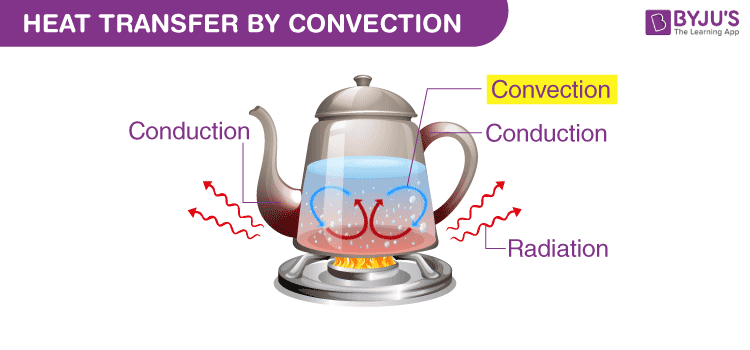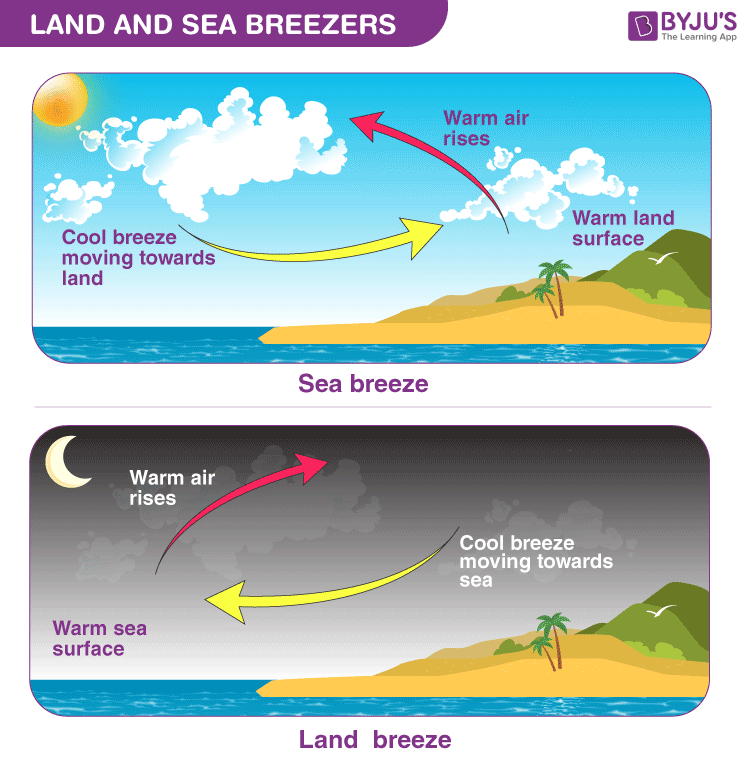# Heat Transfer By Convection

You may have noticed that if you leave a cup of hot coffee on a table, it gets cold in some time. This is because heat is lost to the surroundings. Thus, heat transfer is the transfer of heat or thermal energy between physical systems. So when there is a temperature difference between two bodies, heat is transferred from the hot body to the colder body. There are three common modes of heat transfer – conduction, convection, and radiation. In this article, we will discuss heat transfer through convection.## What is Convection?

Convection is the process of heat transfer by the bulk movement of molecules within fluids such as gases and liquids. The initial heat transfer between the object and the fluid takes place through conduction, but the bulk heat transfer happens due to the motion of the fluid.

• Convection is the process of heat transfer in fluids by the actual motion of matter.
• It happens in liquids and gases.
• It may be natural or forced.
• It involves a bulk transfer of portions of the fluid.

### How is Heat Transferred through Convection?

When a fluid is heated from below, thermal expansion takes place. The lower layers of the fluid, which are hotter, become less dense. We know that colder fluid is denser. Due to buoyancy, the less dense, hotter part of the fluid rises up. And the colder, denser fluid replaces it. This process is repeated when this part also gets heated and rises up to be replaced by the colder upper layer. This is how the heat is transferred through convection.

## Types of Convection

There are two types of convection, and they are:

• Natural convection
• Forced convection

Natural convection: When convection takes place due to buoyant force as there is a difference in densities caused by the difference in temperatures it is known as natural convection.

Examples of natural convection are oceanic winds.

Forced convection: When external sources such as fans and pumps are used for creating induced convection, it is known as forced convection.

Examples of forced convection are using water heaters or geysers for instant heating of water and using a fan on a hot summer day.

Forced convection is related to Newton’s law of cooling, which is given as:

 $$\begin{array}{l}P = \frac{dQ}{dt}=hA(T-T_{0})\end{array}$$

Where,

• $$\begin{array}{l}P = \frac{dQ}{dt}\end{array}$$
is the rate at which heat is transferred
• h is the convection heat-transfer coefficient
• A is the exposed surface area
• T is the temperature of the immersed object
• T0 is the temperature of the fluid which is under convection

The value of the heat-transfer coefficient h depends on:

• Density
• Viscosity
• Thermal conductivity
• Specific heat capacity

To know more about convection methods of heat transfer in detail, click on the video below.## Convection Examples

As we have read previously, convection can either be forced or natural. One of the most common examples of natural convection is the phenomena of the sea and land breeze.

• Sea breeze: This phenomenon occurs during the day. The sun heats up both the sea surface and land. As the sea has a greater heating capacity, it absorbs much of the sun’s energy but gets warmed up much slower than the land. As a result, the temperature above the land rises and heats the air in the atmosphere above it. Warm air is less dense, and hence, it expands, creating a low-pressure area over the land near the coast. Meanwhile, there is relatively high pressure over the sea. The difference in air pressure causes the air to flow from sea to land. The sudden gush of wind felt due to this is known as the sea breeze.
• Land Breeze: This phenomenon occurs during the night when the situation reverses. As the sun sets, the land and sea start cooling down. The land quickly loses heat when compared to water due to the differences in heat capacity. Consequently, the temperature of the sea is relatively higher, which creates low air pressure there. This sets up a flow of cool breeze offshore, known as the land breeze.The examples of forced convection include a water pump in an automobile engine, suction devices, forced air heating systems, etc.## Frequently Asked Questions – FAQs

Q1

### State heat definition

According to the heat definition, heat is a form of energy that can be transferred from one medium to another through various processes like conduction, convection and radiation.
Q2

### What is convection?

Convection is the process of heat transfer by the bulk movement of molecules within fluids such as gases and liquids.
Q3

### On what factors the heat-transfer coefficient (h) depends upon?

The value of heat-transfer coefficient (h) depends on:

• Density
• Viscosity
• Thermal conductivity
• Specific heat capacity
• Q4

TRUE.
Q5

### What are the types of convection?

Types of convection are:
• Natural convection
• Forced convection
• Hope you have understood the heat definition and the process of heat transfer in detail. To know more, visit BYJU’S – The Learning App!

Test your knowledge on Heat transfer - Convection